# Lions Weekly: August 31, 2022# A Note From Mr. Sandefur

Lion Family,

A month of this school year comes to a close today, and it is hard to imagine that we are already quickly approaching a change in season on campus. Though it feels like students began classes yesterday, we are fully aware that we are four weeks smarter in terms of content gained and four weeks wiser in terms of biblical connections, relationships built, etc. As we enter into the fall, it is my prayer that our students and families will work diligently to be the hands and feet of Christ to those we encounter as we seek to lovingly serve one another.

Our “Love Where We Live” service campaign kicks off with enthusiasm this month as our fourth and ninth grades participate in projects that will serve our community. Our LWWL focus allows us to teach our students the importance of serving others as Christ serves us and shows our community the love of Christ. As your students participate in these projects throughout the year, please join us in reinforcing these critical beliefs at home.

In Christ Alone,

Jeremy Sandefur

 table div table+table+table+table+table div table{width:100%;padding:0}table div table+table+table+table+table div table img{width:96.23%;padding:0;float:none}table div table+table+table+table+table div table td{width:100%;padding:0 1.88% 18px}/* styles */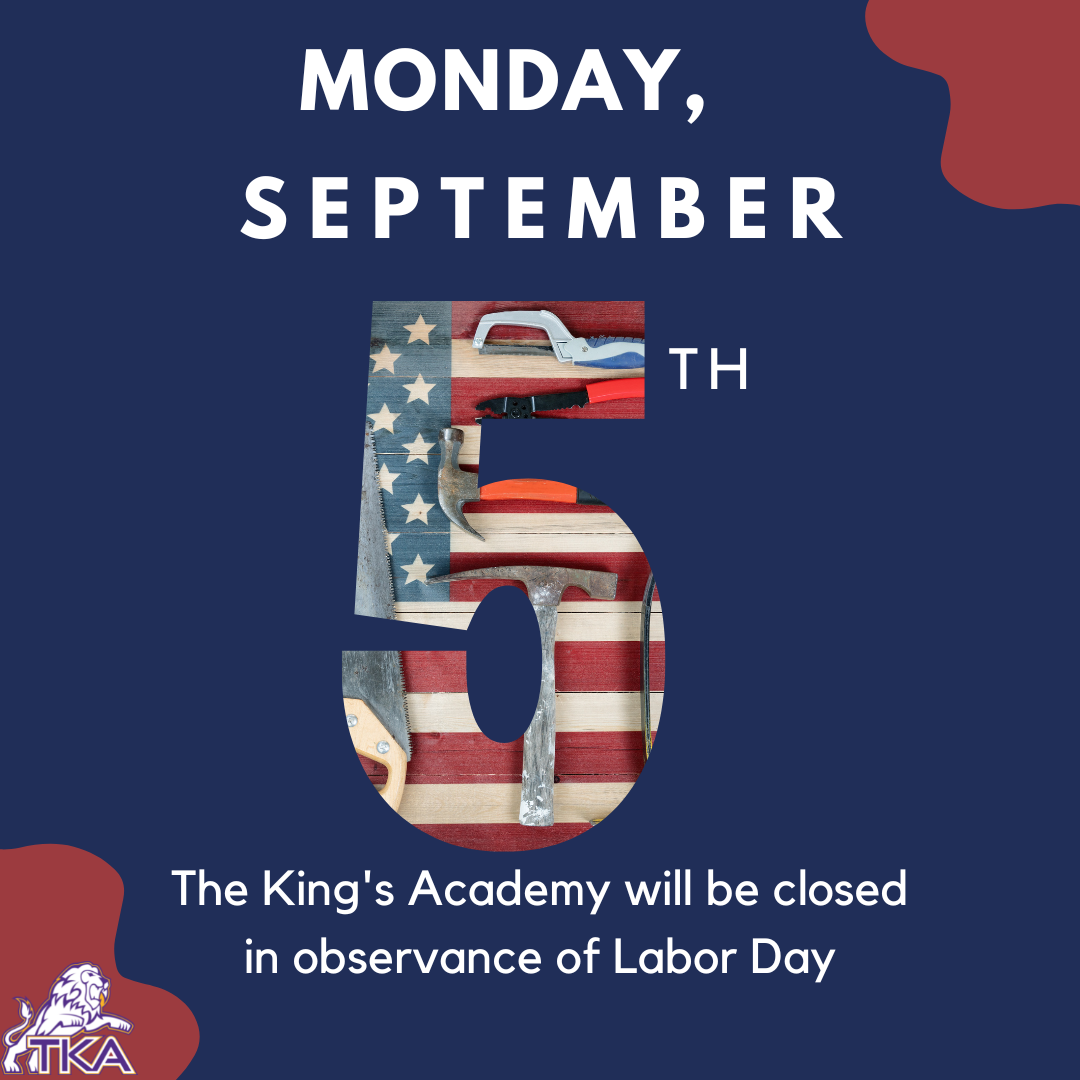# Labor Day

TKA school and offices will be closed on Monday, September 5, in observance of Labor Day.

 table div table+table+table+table+table+table+table div table{width:100%;padding:0}table div table+table+table+table+table+table+table div table img{width:96.23%;padding:0;float:none}table div table+table+table+table+table+table+table div table td{width:100%;padding:0 1.88% 18px}/* styles */# Grandparents' Day 2022

We are extremely excited to share with you that Grandparents’ Day is returning to TKA this fall on Friday, September 23, 2022. We understand the importance of sharing these integral moments between child and grandparents to create lasting memories. We are thrilled to welcome back all grandparents to TKA for this special event.

Grandparents are invited to arrive with their grandchild(ren) at 7:45 a.m. to check in with their little lion’s teacher in the classroom to enjoy coffee, refreshments, fellowship, and an activity. After time in the classroom, grandparents are invited to attend our Grandparents’ Day Program hosted in TKA Tibbetts Gymnasium. We ask for our grandparents to arrive in Tibbetts by 8:40 a.m. to receive a token of our appreciation for your dedication and support for TKA. Any middle/high school sibling may also attend the program with their grandparents. These students must be given permission on the form below to be dismissed from class. The Grandparents’ Day Program will include our elementary student body leading in worship, our HS Chapel Band, a song by our MS/HS Choir, followed by a word of encouragement from a Guest Speaker. Following the program, students who are given parent permission via the Student Release Form, will be allowed to dismiss with their grandparents. Grandparents are encouraged to visit our Book Fair and tour the campus before they leave. These opportunities will be available until 12:00 p.m. to allow our remaining students to continue their normal academic schedule.

Please RSVP on the link below by Friday, September 16th. This link will also be used for parent permission for student release. The student release will allow a student’s grandparent to check them out of school following the program. On the day of the event, grandparents will sign out your child in the classroom with your child’s teacher, not the elementary office.

 /* styles */ If you have any questions, please contact me at ksullivan@thekingsacademy.net. We are looking forward to a wonderful time of fellowship with all grandparents. Blessings, Kelly Sullivan, Elementary Principal
 table div table+table+table+table+table+table+table+table+table+table+table div table{width:100%;padding:0}table div table+table+table+table+table+table+table+table+table+table+table div table img{width:96.23%;padding:0;float:none}table div table+table+table+table+table+table+table+table+table+table+table div table td{width:100%;padding:0 1.88% 18px}/* styles */# Welcome to TKA

On behalf of the Leadership Team of The King's Academy, we are excited to announce Mrs.Julie DeBusk as our new High School History instructor. Julie comes to us with a wealth of experience in Christian Education as she begins her 29th year in education.

 table div table+table+table+table+table+table+table+table+table+table+table+table+table div table{width:100%;padding:0}table div table+table+table+table+table+table+table+table+table+table+table+table+table div table img{width:96.23%;padding:0;float:none}table div table+table+table+table+table+table+table+table+table+table+table+table+table div table td{width:100%;padding:0 1.88% 18px}/* styles */# Jr. and Sr. College Trip

Our Junior and Senior college road trip is just around the corner!

Final payments and permission slips are due to Mrs. Griffin by Friday, September 2.

 table div table+table+table+table+table+table+table+table+table+table+table+table+table+table+table div table{width:100%;padding:0}table div table+table+table+table+table+table+table+table+table+table+table+table+table+table+table div table img{width:96.23%;padding:0;float:none}table div table+table+table+table+table+table+table+table+table+table+table+table+table+table+table div table td{width:100%;padding:0 1.88% 18px}/* styles */# The Lion's Den

The Lion's Den pop-up store will be in a few locations this week for you to purchase some TKA spirit items.

▪ Thursday, September 1, from 5:00 p.m. - 7:00 p.m. in front of Tibbetts during the high school Volleyball game.
▪ Friday, September 2, from
7:00 p.m. to 10:00 p.m. outside of Stokley during the football game.
 ▪ Thursday, September 1, from 5:00 p.m. - 7:00 p.m. in front of Tibbetts during the high school Volleyball game.
 ▪ Friday, September 2, from 7:00 p.m. to 10:00 p.m. outside of Stokley during the football game.

Stop by and shop at these different times and locations!

 table div table+table+table+table+table+table+table+table+table+table+table+table+table+table+table+table+table div table{width:100%;padding:0}table div table+table+table+table+table+table+table+table+table+table+table+table+table+table+table+table+table div table img{width:96.23%;padding:0;float:none}table div table+table+table+table+table+table+table+table+table+table+table+table+table+table+table+table+table div table td{width:100%;padding:0 1.88% 18px}/* styles */# PTF Coffee Mingle

PTF would like to invite members and those who would like to join PTF for coffee and conversation. So, mark your calendars and save the date for the PTF Coffee Mingle on Friday, September 23, at The Bridge Coffee House.

 table div table+table+table+table+table+table+table+table+table+table+table+table+table+table+table+table+table+table+table div table{width:100%;padding:0}table div table+table+table+table+table+table+table+table+table+table+table+table+table+table+table+table+table+table+table div table img{width:96.23%;padding:0;float:none}table div table+table+table+table+table+table+table+table+table+table+table+table+table+table+table+table+table+table+table div table td{width:100%;padding:0 1.88% 18px}/* styles */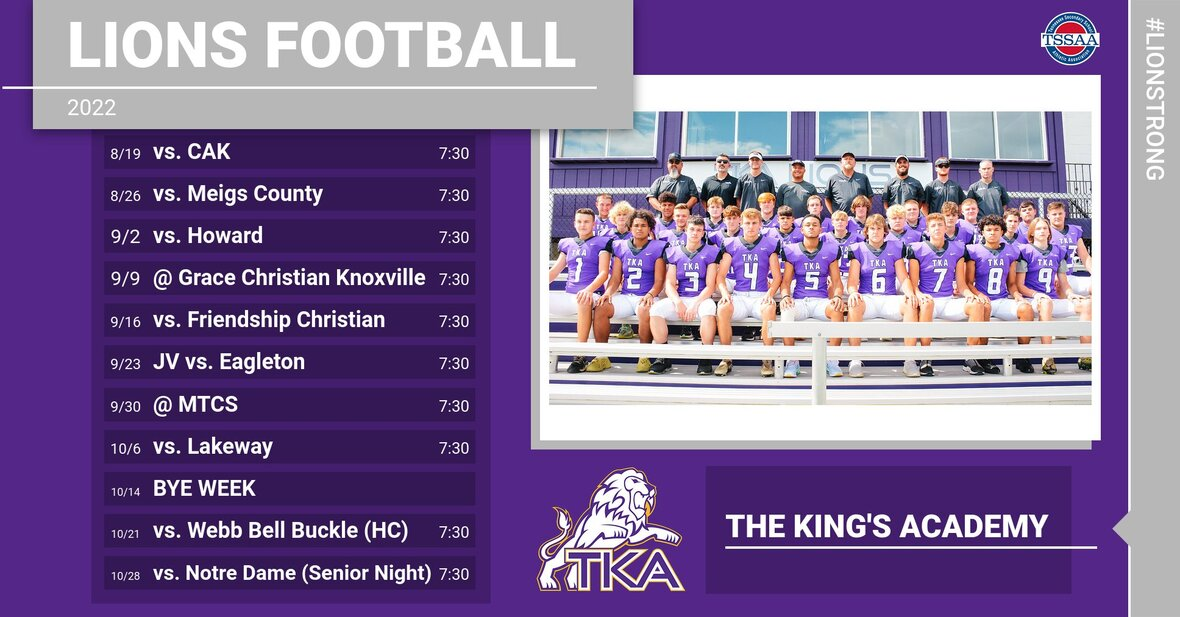# Athletic Event Tickets

This Friday is our second season football game! Mark your calendars and make plans to come cheer our Lions to victory! Below are a few updates for this season’s game tickets and parking passes:

▪ ALL TICKETS WILL BE SOLD ON GOFAN WE WILL NOT SELL PAPER TICKETS
▪ To purchase tickets on GoFan, use this link.
▪ All TKA students and staff members are free.
▪ All up-to-date TSSAA passes will be accepted.
▪ Individual season tickets are available for adults for \$60, students for \$35.
▪ Adult individual season tickets + parking pass available for \$95.
▪ Individual season parking passes available for \$40.
▪ Tickets are \$8 for adults, \$5 for students, kids 5 and under get in free.
▪ Parking is \$5 and parking passes are available on GoFan. If you buy your ticket through Go Fan, you may park in any of the four parking lots (Stokely (Pre-paid ONLY), Cartee (Pre-paid ONLY), Tibbetts, or Visitors). If you purchase parking upon arrival, the cost is \$5 but cash will ONLY be taken at the Tibbetts or Visitor lots.
▪ Free parking is available at Ogle and at the Weekly Softball Field.
▪ Gates will open at 6:00 p.m.
▪ Kickoff is at 7:30 p.m.
▪ Concessions will be available for purchase with cash or card.
 ▪ ALL TICKETS WILL BE SOLD ON GOFAN WE WILL NOT SELL PAPER TICKETS
 ▪ To purchase tickets on GoFan, use this link.
 ▪ All TKA students and staff members are free.
 ▪ All up-to-date TSSAA passes will be accepted.
 ▪ Individual season tickets are available for adults for \$60, students for \$35.
 ▪ Adult individual season tickets + parking pass available for \$95.
 ▪ Individual season parking passes available for \$40.
 ▪ Tickets are \$8 for adults, \$5 for students, kids 5 and under get in free.
 ▪ Parking is \$5 and parking passes are available on GoFan. If you buy your ticket through Go Fan, you may park in any of the four parking lots (Stokely (Pre-paid ONLY), Cartee (Pre-paid ONLY), Tibbetts, or Visitors). If you purchase parking upon arrival, the cost is \$5 but cash will ONLY be taken at the Tibbetts or Visitor lots.
 ▪ Free parking is available at Ogle and at the Weekly Softball Field.
 ▪ Gates will open at 6:00 p.m.
 ▪ Kickoff is at 7:30 p.m.
 ▪ Concessions will be available for purchase with cash or card.

See you Friday night at Huskey field!

 table div table+table+table+table+table+table+table+table+table+table+table+table+table+table+table+table+table+table+table+table+table+table div table{width:100%;padding:0}table div table+table+table+table+table+table+table+table+table+table+table+table+table+table+table+table+table+table+table+table+table+table div table img{width:96.23%;padding:0;float:none}table div table+table+table+table+table+table+table+table+table+table+table+table+table+table+table+table+table+table+table+table+table+table div table td{width:100%;padding:0 1.88% 18px}/* styles */# Dress Up Day: Constructing Our Way to Victory!

This Friday, September 2, dress in construction attire as we construct a championship season!

Remember, all attire must adhere to dress code.

# Fall 2022 Dress Up Day Themes

Remember, all attire must adhere to dress code

▪ Friday, September 16 - Hunting for a Repeat (Dress in Camo)
▪ Friday, September 23 - Gold Out (Dress in Gold for Childhood Cancer Awareness)
▪ Friday, October 7 - Pink Out (Dress in Pink for Breast Cancer Awareness)
▪ Tuesday, October 18 - Friday, October 21 - Homecoming Week (see details below)
▪ Friday, October 28 - "Senior" Spirit Day (Dress in "Senior" clothes in honor of Senior night)
 ▪ Friday, September 16 - Hunting for a Repeat (Dress in Camo)
 ▪ Friday, September 23 - Gold Out (Dress in Gold for Childhood Cancer Awareness)
 ▪ Friday, October 7 - Pink Out (Dress in Pink for Breast Cancer Awareness)
 ▪ Tuesday, October 18 - Friday, October 21 - Homecoming Week (see details below)
 ▪ Friday, October 28 - "Senior" Spirit Day (Dress in "Senior" clothes in honor of Senior night)

## Homecoming Week: Blast from the Past

▪ Tuesday, October 18 - 90s Dress Up Day (Dress in 90s attire from movies, TV shows, musicians, or sports stars)
▪ Wednesday, October 19 - 80s Dress Up Day (Dress in 80s attire from movies, TV shows, musicians, or sports stars)
▪ Thursday, October 20 - 70s Dress Up Day (Dress in 70s attire from movies, TV shows, musicians, or sports stars)
▪ Friday, October 21 - Homecoming T-shirt or TKA Spirit attire
 ▪ Tuesday, October 18 - 90s Dress Up Day (Dress in 90s attire from movies, TV shows, musicians, or sports stars)
 ▪ Wednesday, October 19 - 80s Dress Up Day (Dress in 80s attire from movies, TV shows, musicians, or sports stars)
 ▪ Thursday, October 20 - 70s Dress Up Day (Dress in 70s attire from movies, TV shows, musicians, or sports stars)
 ▪ Friday, October 21 - Homecoming T-shirt or TKA Spirit attire
 table div table+table+table+table+table+table+table+table+table+table+table+table+table+table+table+table+table+table+table+table+table+table+table+table+table+table div table{width:100%;padding:0}table div table+table+table+table+table+table+table+table+table+table+table+table+table+table+table+table+table+table+table+table+table+table+table+table+table+table div table img{width:96.23%;padding:0;float:none}table div table+table+table+table+table+table+table+table+table+table+table+table+table+table+table+table+table+table+table+table+table+table+table+table+table+table div table td{width:100%;padding:0 1.88% 18px}/* styles */# Last Day to Order

PTF is selling 12" purple and yellow mums for \$18 each through this evening.

The girl or boy with the most mum sales will be the crown and ball holder at the Homecoming game on Friday, October 21.

Don't miss out on these mums! Click the link below to order yours today!

Mums will be available for pick up on Thursday, September 8 from 2PM-7PM at the soccer field by Ogle Building.

 table div table+table+table+table+table+table+table+table+table+table+table+table+table+table+table+table+table+table+table+table+table+table+table+table+table+table+table+table+table div table{width:100%;padding:0}table div table+table+table+table+table+table+table+table+table+table+table+table+table+table+table+table+table+table+table+table+table+table+table+table+table+table+table+table+table div table img{width:96.23%;padding:0;float:none}table div table+table+table+table+table+table+table+table+table+table+table+table+table+table+table+table+table+table+table+table+table+table+table+table+table+table+table+table+table div table td{width:100%;padding:0 1.88% 18px}/* styles */# Choir Highlight

Three of our High School Honors Choir students (Brelan Delius, Ezequiel Jose, and Chloe Shirley) attended the All-East/All-State Preparatory Clinic at UT Knoxville this past Saturday, August 27th. They will be competing for a spot in the All-East Choirs very soon. Way to go Lions!

 table div table+table+table+table+table+table+table+table+table+table+table+table+table+table+table+table+table+table+table+table+table+table+table+table+table+table+table+table+table+table+table div table{width:100%;padding:0}table div table+table+table+table+table+table+table+table+table+table+table+table+table+table+table+table+table+table+table+table+table+table+table+table+table+table+table+table+table+table+table div table img{width:96.23%;padding:0;float:none}table div table+table+table+table+table+table+table+table+table+table+table+table+table+table+table+table+table+table+table+table+table+table+table+table+table+table+table+table+table+table+table div table td{width:100%;padding:0 1.88% 18px}/* styles */# Daily Announcements

## Elementary

Did you know that you can listen to Ms. Sullivan's weekly announcements? Open the TKA app and go to "elementary". Then, click "link list" followed by clicking on "TKA Elementary Site by Principal Sullivan". There you will find a link to Ms. Sullivan's announcements under the Weekly Announcement tab.

## Middle and High

Did you know that you can listen to Mr. Fuller's morning announcements in the app? Simply open the app and go to "watch". There you will find a link to Mr. Fuller's daily announcements for middle and high schoolers.

 table div table+table+table+table+table+table+table+table+table+table+table+table+table+table+table+table+table+table+table+table+table+table+table+table+table+table+table+table+table+table+table+table+table div table{width:100%;padding:0}table div table+table+table+table+table+table+table+table+table+table+table+table+table+table+table+table+table+table+table+table+table+table+table+table+table+table+table+table+table+table+table+table+table div table img{width:96.23%;padding:0;float:none}table div table+table+table+table+table+table+table+table+table+table+table+table+table+table+table+table+table+table+table+table+table+table+table+table+table+table+table+table+table+table+table+table+table div table td{width:100%;padding:0 1.88% 18px}/* styles */# Elementary Fall Clubs

TKA Elementary Families,

We are excited to announce that our TKA Elementary club opportunities will begin very soon. Each club will have a fall section and a spring section.

The fall section will begin the week of September 12th and end December 9th. The spring section will begin the week of January 30th and end April 21st. Registration for the spring sections will be shared second semester.

Each club will include a \$20 participation fee to cover resources needed for club activities. Each club has a maximum number of 15 students.

Please click on a club to review the club description and registration link. We will select the first participants until the maximum number is reached. We ask that you consider all options and register for clubs that fit your schedule for the year. To be fair to our club instructors, we ask that you choose a club where your child will be able to participate and attend 90% of the semester club activities.

All sign up forms will close Friday, September 2nd. Our club teachers will be in communication with participant families for their club area by Friday, September 9th. This communication will include the next steps prior to the first meeting the following week.

We are very blessed to have such wonderful teachers who are excited to provide these enriching opportunities after school hours to further enhance our elementary learning.

Blessings,
Kelly Sullivan
Elementary Principal

 table div table+table+table+table+table+table+table+table+table+table+table+table+table+table+table+table+table+table+table+table+table+table+table+table+table+table+table+table+table+table+table+table+table+table+table div table{width:100%;padding:0}table div table+table+table+table+table+table+table+table+table+table+table+table+table+table+table+table+table+table+table+table+table+table+table+table+table+table+table+table+table+table+table+table+table+table+table div table img{width:96.23%;padding:0;float:none}table div table+table+table+table+table+table+table+table+table+table+table+table+table+table+table+table+table+table+table+table+table+table+table+table+table+table+table+table+table+table+table+table+table+table+table div table td{width:100%;padding:0 1.88% 18px}/* styles */Book Club will provide students with the opportunity to engage with texts deeply in a relaxed fun environment. Through group discussion and hands-on activities, students will practice age appropriate comprehension skills such as character analysis, distinguishing literary themes, understanding the elements of plot development, and using textual evidence. Book club will also provide time for readers to share book recommendations with each other, through book talks and book lists, in order to deepen their self-knowledge as a reader and broaden their range of choice in reading material. Reading choice is vital to developing a lifelong love of reading. Choice of club texts will be determined in the first few meetings of the club so readers can have input and ownership of their experience.

 table div table+table+table+table+table+table+table+table+table+table+table+table+table+table+table+table+table+table+table+table+table+table+table+table+table+table+table+table+table+table+table+table+table+table+table+table+table+table+table div table{width:100%;padding:0}table div table+table+table+table+table+table+table+table+table+table+table+table+table+table+table+table+table+table+table+table+table+table+table+table+table+table+table+table+table+table+table+table+table+table+table+table+table+table+table div table img{width:96.23%;padding:0;float:none}table div table+table+table+table+table+table+table+table+table+table+table+table+table+table+table+table+table+table+table+table+table+table+table+table+table+table+table+table+table+table+table+table+table+table+table+table+table+table+table div table td{width:100%;padding:0 1.88% 18px}/* styles */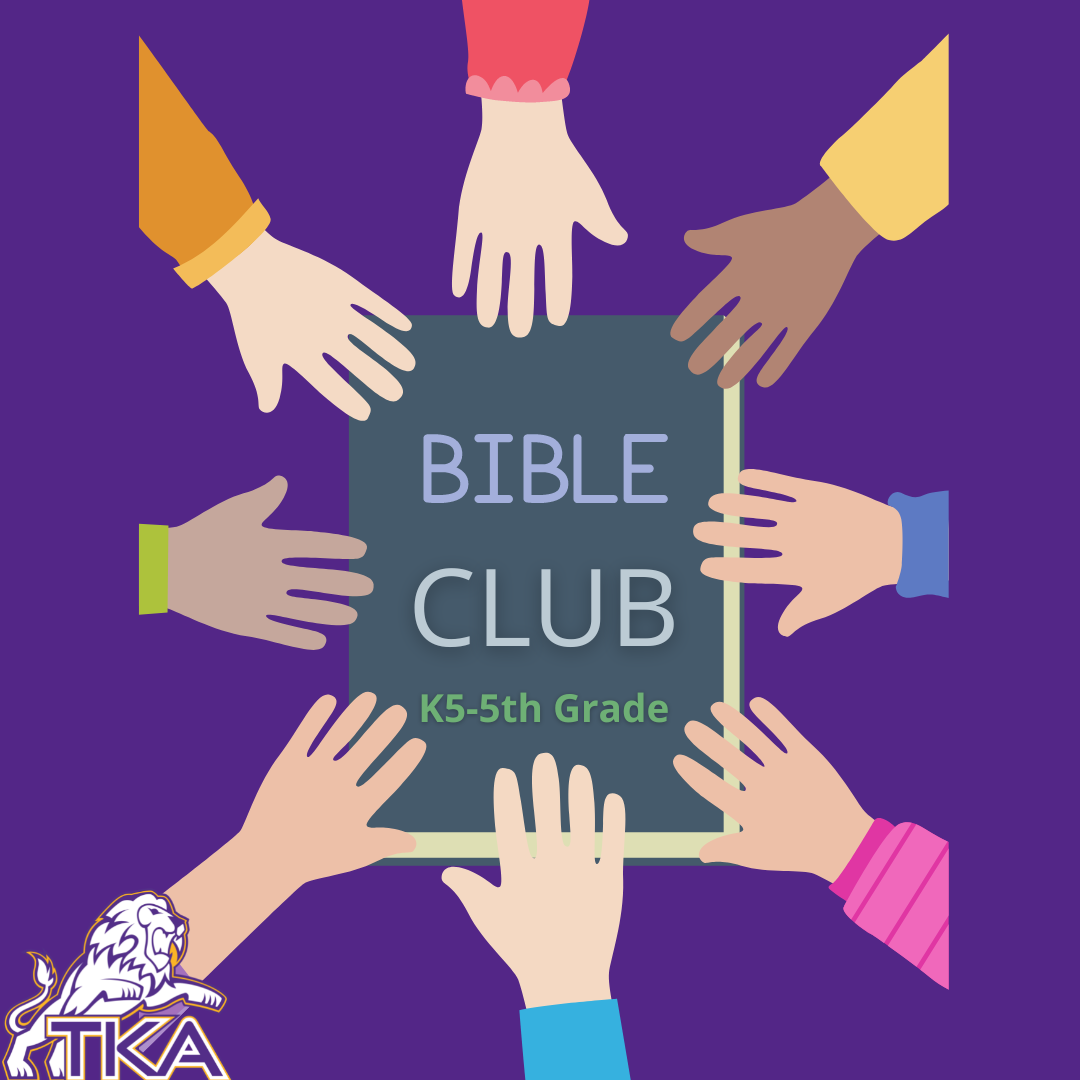Bible Club will include Bible Drill, Memory Verses, Bible Story Interacting (drawing, drama, games, questions, songs,) . . . and Developing a Personal Quiet Time.

 table div table+table+table+table+table+table+table+table+table+table+table+table+table+table+table+table+table+table+table+table+table+table+table+table+table+table+table+table+table+table+table+table+table+table+table+table+table+table+table+table+table+table+table div table{width:100%;padding:0}table div table+table+table+table+table+table+table+table+table+table+table+table+table+table+table+table+table+table+table+table+table+table+table+table+table+table+table+table+table+table+table+table+table+table+table+table+table+table+table+table+table+table+table div table img{width:96.23%;padding:0;float:none}table div table+table+table+table+table+table+table+table+table+table+table+table+table+table+table+table+table+table+table+table+table+table+table+table+table+table+table+table+table+table+table+table+table+table+table+table+table+table+table+table+table+table+table div table td{width:100%;padding:0 1.88% 18px}/* styles */# K4-K5 STEAM Club

K4-K5 STEAM Club will include a variety of hands-on activities focusing on science, technology, engineering, arts, and mathematics that are age appropriate for our little lions.

 table div table+table+table+table+table+table+table+table+table+table+table+table+table+table+table+table+table+table+table+table+table+table+table+table+table+table+table+table+table+table+table+table+table+table+table+table+table+table+table+table+table+table+table+table+table+table div table{width:100%;padding:0}table div table+table+table+table+table+table+table+table+table+table+table+table+table+table+table+table+table+table+table+table+table+table+table+table+table+table+table+table+table+table+table+table+table+table+table+table+table+table+table+table+table+table+table+table+table+table div table img{width:96.23%;padding:0;float:none}table div table+table+table+table+table+table+table+table+table+table+table+table+table+table+table+table+table+table+table+table+table+table+table+table+table+table+table+table+table+table+table+table+table+table+table+table+table+table+table+table+table+table+table+table+table+table div table td{width:100%;padding:0 1.88% 18px}/* styles */# STEAM Club (1-2 grades only)

This STEAM Club will include a variety of hands-on activities focusing on science, technology, engineering, arts, and mathematics that are age appropriate for our primary lions.

 table div table+table+table+table+table+table+table+table+table+table+table+table+table+table+table+table+table+table+table+table+table+table+table+table+table+table+table+table+table+table+table+table+table+table+table+table+table+table+table+table+table+table+table+table+table+table+table+table+table div table{width:100%;padding:0}table div table+table+table+table+table+table+table+table+table+table+table+table+table+table+table+table+table+table+table+table+table+table+table+table+table+table+table+table+table+table+table+table+table+table+table+table+table+table+table+table+table+table+table+table+table+table+table+table+table div table img{width:96.23%;padding:0;float:none}table div table+table+table+table+table+table+table+table+table+table+table+table+table+table+table+table+table+table+table+table+table+table+table+table+table+table+table+table+table+table+table+table+table+table+table+table+table+table+table+table+table+table+table+table+table+table+table+table+table div table td{width:100%;padding:0 1.88% 18px}/* styles */# Whiz Kidz (3-5 grades only)

This STEAM Club will include a variety of hands-on activities focusing on science, technology, engineering, arts, and mathematics that are age appropriate for our intermediate lions.

 table div table+table+table+table+table+table+table+table+table+table+table+table+table+table+table+table+table+table+table+table+table+table+table+table+table+table+table+table+table+table+table+table+table+table+table+table+table+table+table+table+table+table+table+table+table+table+table+table+table+table+table+table div table{width:100%;padding:0}table div table+table+table+table+table+table+table+table+table+table+table+table+table+table+table+table+table+table+table+table+table+table+table+table+table+table+table+table+table+table+table+table+table+table+table+table+table+table+table+table+table+table+table+table+table+table+table+table+table+table+table+table div table img{width:96.23%;padding:0;float:none}table div table+table+table+table+table+table+table+table+table+table+table+table+table+table+table+table+table+table+table+table+table+table+table+table+table+table+table+table+table+table+table+table+table+table+table+table+table+table+table+table+table+table+table+table+table+table+table+table+table+table+table+table div table td{width:100%;padding:0 1.88% 18px}/* styles */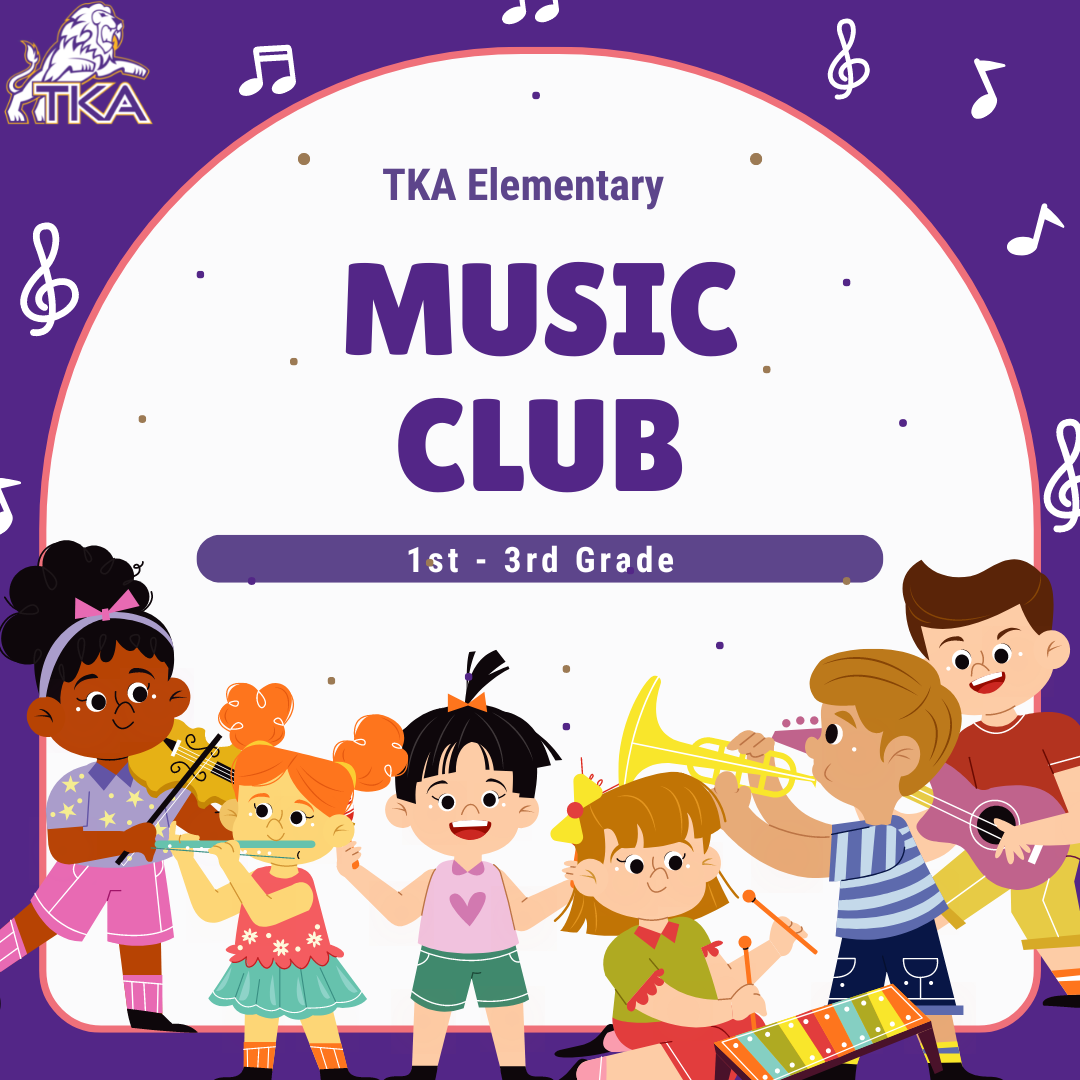# Music Club (Grades 1-3 only)

Join us as we Create, Perform, and Respond to music in lots of fun ways! A few of the activities we will be doing:

▪ Singing
▪ Playing instruments
▪ Moving to music
▪ Listening to music
▪ Composing, arranging, and performing music
 ▪ Singing
 ▪ Playing instruments
 ▪ Moving to music
 ▪ Listening to music
 ▪ Composing, arranging, and performing music
 table div table+table+table+table+table+table+table+table+table+table+table+table+table+table+table+table+table+table+table+table+table+table+table+table+table+table+table+table+table+table+table+table+table+table+table+table+table+table+table+table+table+table+table+table+table+table+table+table+table+table+table+table+table+table+table div table{width:100%;padding:0}table div table+table+table+table+table+table+table+table+table+table+table+table+table+table+table+table+table+table+table+table+table+table+table+table+table+table+table+table+table+table+table+table+table+table+table+table+table+table+table+table+table+table+table+table+table+table+table+table+table+table+table+table+table+table+table div table img{width:96.23%;padding:0;float:none}table div table+table+table+table+table+table+table+table+table+table+table+table+table+table+table+table+table+table+table+table+table+table+table+table+table+table+table+table+table+table+table+table+table+table+table+table+table+table+table+table+table+table+table+table+table+table+table+table+table+table+table+table+table+table+table div table td{width:100%;padding:0 1.88% 18px}/* styles */# 2021-22 Yearbooks

Preordered yearbooks, are in and delivered to students. If you did not order a yearbook for 2021-22, they can be purchased for \$55 in either school office or by emailing Mr. Josh Smith jsmith@thekingsacademy.net.

 table div table+table+table+table+table+table+table+table+table+table+table+table+table+table+table+table+table+table+table+table+table+table+table+table+table+table+table+table+table+table+table+table+table+table+table+table+table+table+table+table+table+table+table+table+table+table+table+table+table+table+table+table+table+table+table+table+table div table{width:100%;padding:0}table div table+table+table+table+table+table+table+table+table+table+table+table+table+table+table+table+table+table+table+table+table+table+table+table+table+table+table+table+table+table+table+table+table+table+table+table+table+table+table+table+table+table+table+table+table+table+table+table+table+table+table+table+table+table+table+table+table div table img{width:96.23%;padding:0;float:none}table div table+table+table+table+table+table+table+table+table+table+table+table+table+table+table+table+table+table+table+table+table+table+table+table+table+table+table+table+table+table+table+table+table+table+table+table+table+table+table+table+table+table+table+table+table+table+table+table+table+table+table+table+table+table+table+table+table div table td{width:100%;padding:0 1.88% 18px}/* styles */# 9-12th Spring Break Trip: France, Germany and The Alps

## March 11-18, 2023

 table div table+table+table+table+table+table+table+table+table+table+table+table+table+table+table+table+table+table+table+table+table+table+table+table+table+table+table+table+table+table+table+table+table+table+table+table+table+table+table+table+table+table+table+table+table+table+table+table+table+table+table+table+table+table+table+table+table+table+table div table{width:100%;padding:0}table div table+table+table+table+table+table+table+table+table+table+table+table+table+table+table+table+table+table+table+table+table+table+table+table+table+table+table+table+table+table+table+table+table+table+table+table+table+table+table+table+table+table+table+table+table+table+table+table+table+table+table+table+table+table+table+table+table+table+table div table img{width:96.23%;padding:0;float:none}table div table+table+table+table+table+table+table+table+table+table+table+table+table+table+table+table+table+table+table+table+table+table+table+table+table+table+table+table+table+table+table+table+table+table+table+table+table+table+table+table+table+table+table+table+table+table+table+table+table+table+table+table+table+table+table+table+table+table+table div table td{width:100%;padding:0 1.88% 18px}/* styles */# Weekly Sports Schedule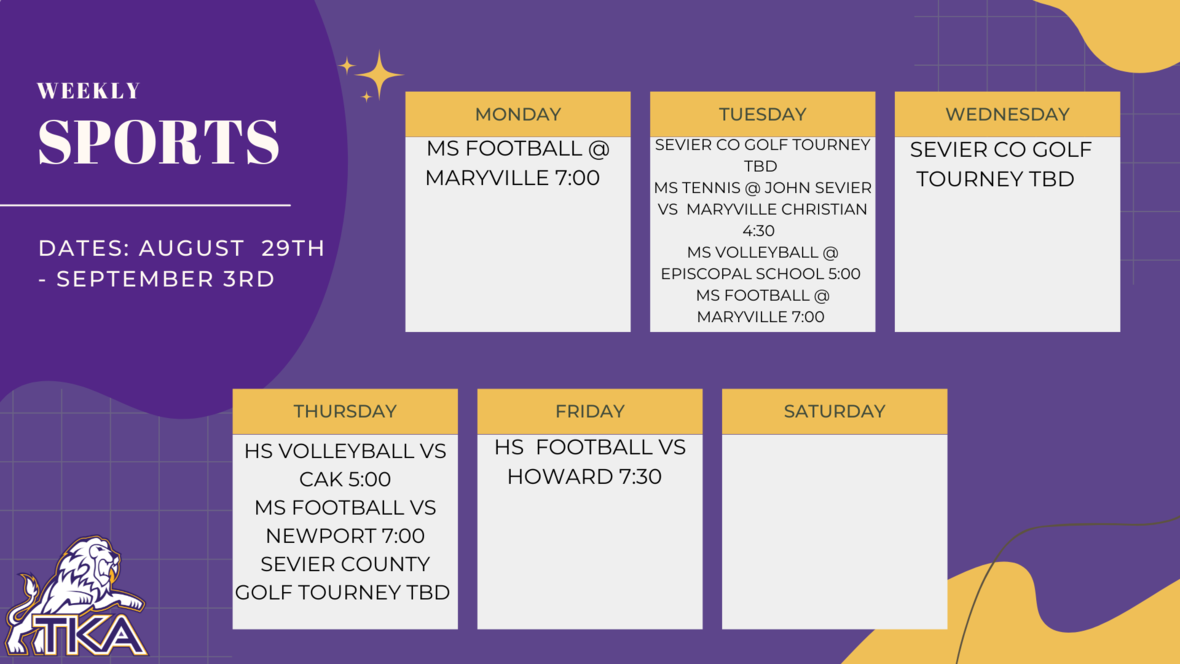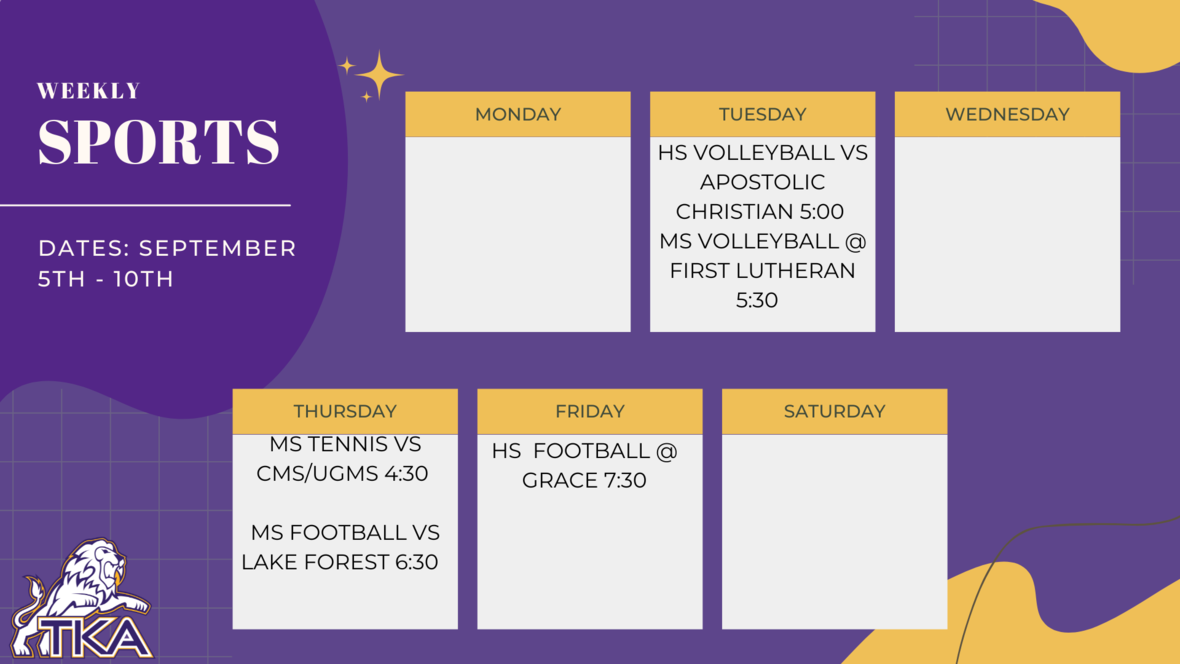table div table+table+table+table+table+table+table+table+table+table+table+table+table+table+table+table+table+table+table+table+table+table+table+table+table+table+table+table+table+table+table+table+table+table+table+table+table+table+table+table+table+table+table+table+table+table+table+table+table+table+table+table+table+table+table+table+table+table+table+table+table+table+table div table{width:100%;padding:0}table div table+table+table+table+table+table+table+table+table+table+table+table+table+table+table+table+table+table+table+table+table+table+table+table+table+table+table+table+table+table+table+table+table+table+table+table+table+table+table+table+table+table+table+table+table+table+table+table+table+table+table+table+table+table+table+table+table+table+table+table+table+table+table div table img{width:96.23%;padding:0;float:none}table div table+table+table+table+table+table+table+table+table+table+table+table+table+table+table+table+table+table+table+table+table+table+table+table+table+table+table+table+table+table+table+table+table+table+table+table+table+table+table+table+table+table+table+table+table+table+table+table+table+table+table+table+table+table+table+table+table+table+table+table+table+table+table div table td{width:100%;padding:0 1.88% 18px}/* styles */# Important information for the 2022-2023 School Year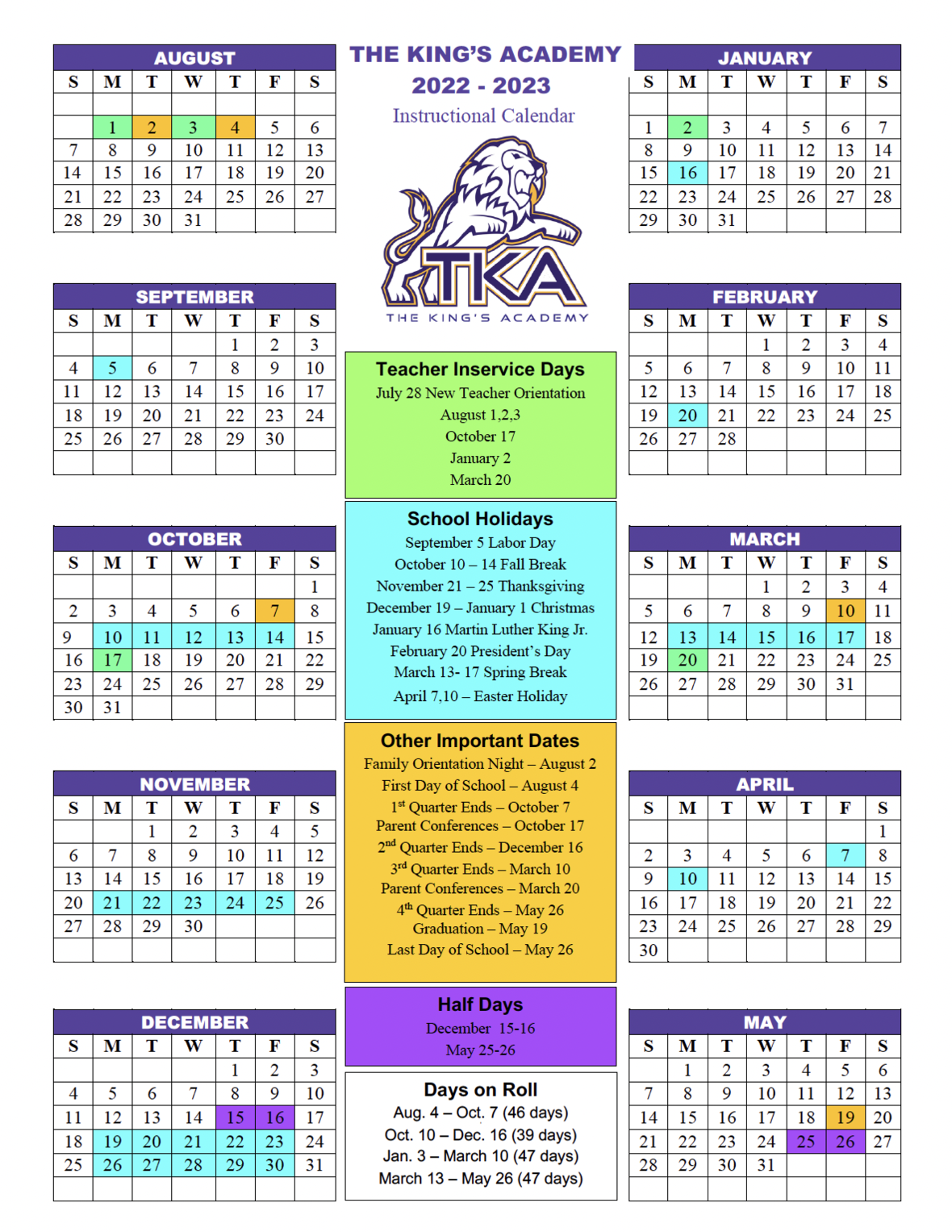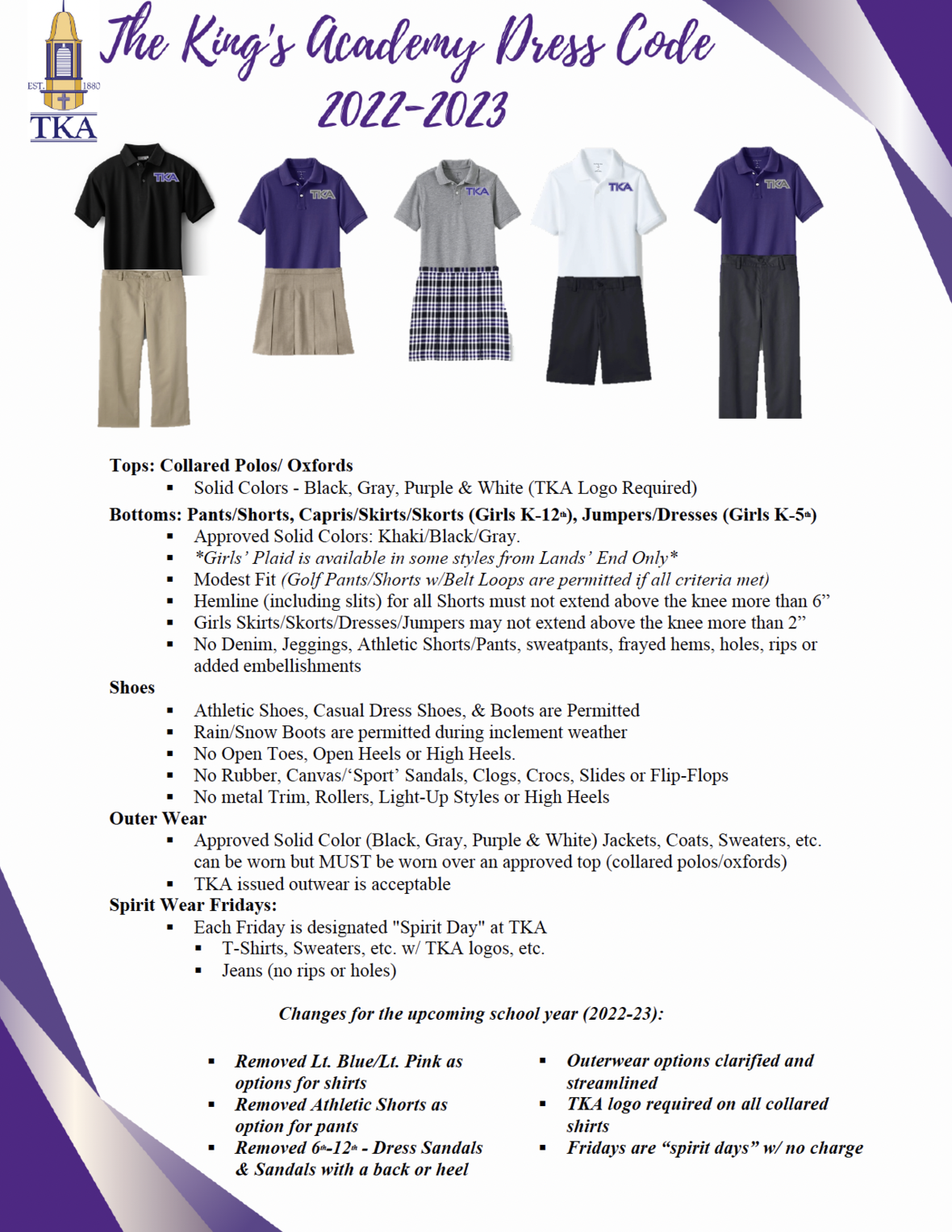table div table+table+table+table+table+table+table+table+table+table+table+table+table+table+table+table+table+table+table+table+table+table+table+table+table+table+table+table+table+table+table+table+table+table+table+table+table+table+table+table+table+table+table+table+table+table+table+table+table+table+table+table+table+table+table+table+table+table+table+table+table+table+table+table+table+table+table div table td,table.module-65{width:100%;padding:0}table div table+table+table+table+table+table+table+table+table+table+table+table+table+table+table+table+table+table+table+table+table+table+table+table+table+table+table+table+table+table+table+table+table+table+table+table+table+table+table+table+table+table+table+table+table+table+table+table+table+table+table+table+table+table+table+table+table+table+table+table+table+table+table+table+table+table+table div table{width:100%;float:none;margin-left:auto;margin-right:auto;padding:0}table div table+table+table+table+table+table+table+table+table+table+table+table+table+table+table+table+table+table+table+table+table+table+table+table+table+table+table+table+table+table+table+table+table+table+table+table+table+table+table+table+table+table+table+table+table+table+table+table+table+table+table+table+table+table+table+table+table+table+table+table+table+table+table+table+table+table+table div table a{border:0 none;text-decoration:none}table div table+table+table+table+table+table+table+table+table+table+table+table+table+table+table+table+table+table+table+table+table+table+table+table+table+table+table+table+table+table+table+table+table+table+table+table+table+table+table+table+table+table+table+table+table+table+table+table+table+table+table+table+table+table+table+table+table+table+table+table+table+table+table+table+table+table+table div table img{width:100%!important;border:0 none;text-decoration:none}/* styles */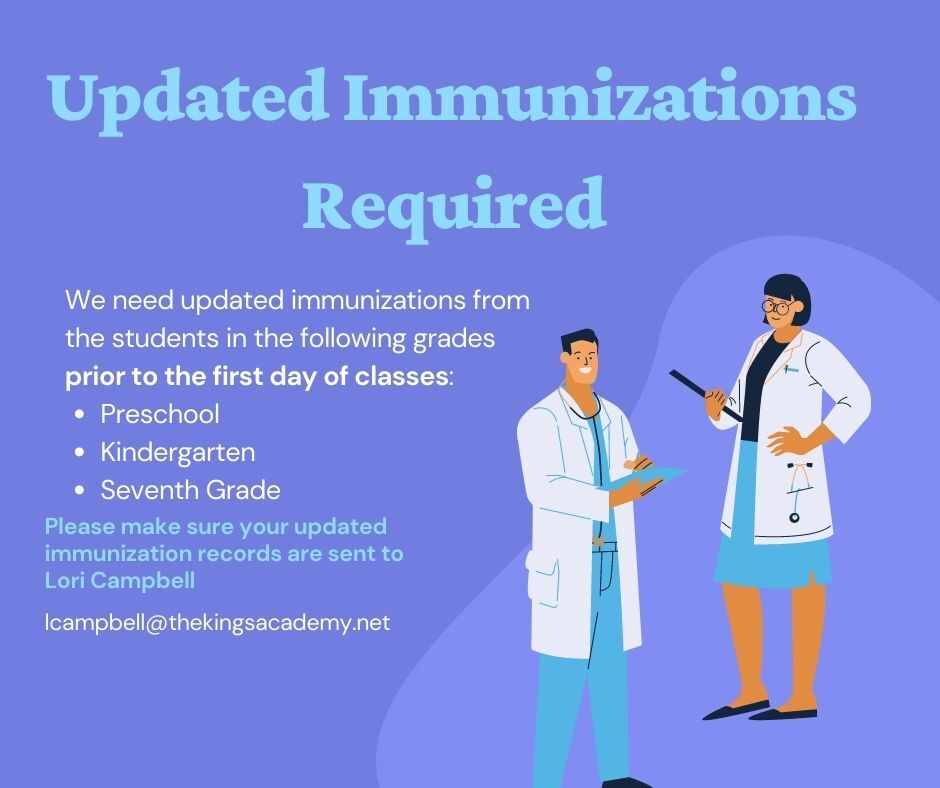table div table+table+table+table+table+table+table+table+table+table+table+table+table+table+table+table+table+table+table+table+table+table+table+table+table+table+table+table+table+table+table+table+table+table+table+table+table+table+table+table+table+table+table+table+table+table+table+table+table+table+table+table+table+table+table+table+table+table+table+table+table+table+table+table+table+table+table+table+table div table{width:100%;padding:0}table div table+table+table+table+table+table+table+table+table+table+table+table+table+table+table+table+table+table+table+table+table+table+table+table+table+table+table+table+table+table+table+table+table+table+table+table+table+table+table+table+table+table+table+table+table+table+table+table+table+table+table+table+table+table+table+table+table+table+table+table+table+table+table+table+table+table+table+table+table div table img{width:96.23%;padding:0;float:none}table div table+table+table+table+table+table+table+table+table+table+table+table+table+table+table+table+table+table+table+table+table+table+table+table+table+table+table+table+table+table+table+table+table+table+table+table+table+table+table+table+table+table+table+table+table+table+table+table+table+table+table+table+table+table+table+table+table+table+table+table+table+table+table+table+table+table+table+table+table div table td{width:100%;padding:0 1.88% 18px}/* styles */# TKA Visitor Policy

In order to help maintain a safe and instructionally based learning environment for our students, all visitors for middle and high school must enter through the front door of the Anderson Building and sign in in the middle/high school office in order to receive a visitor's pass. All visitors for elementary must enter the front door of the Ogle Building in order to receive a visitor's pass. This pass should be visible at all times so that TKA faculty and staff know that you have checked in at the office.

Our doors are open to visitors, but please call ahead of time to schedule conferences with your child’s teachers. This will prevent interruptions to instruction and the loss of the valuable learning time of our students. Adults will not be allowed to wander the halls of the building or hold conferences with teachers during instructional times.

TKA family lunch visitors should follow the above procedure. Lunch tickets are \$5 each.

Students who are not enrolled at TKA are not permitted to visit TKA students during the school day, which includes lunch.

This policy has been developed in order to help maintain a safe and instruction focused environment at school each day. Any visitor who does not meet these expectations may be asked to leave school grounds.

 table div table+table+table+table+table+table+table+table+table+table+table+table+table+table+table+table+table+table+table+table+table+table+table+table+table+table+table+table+table+table+table+table+table+table+table+table+table+table+table+table+table+table+table+table+table+table+table+table+table+table+table+table+table+table+table+table+table+table+table+table+table+table+table+table+table+table+table+table+table+table+table div table{width:100%;padding:0}table div table+table+table+table+table+table+table+table+table+table+table+table+table+table+table+table+table+table+table+table+table+table+table+table+table+table+table+table+table+table+table+table+table+table+table+table+table+table+table+table+table+table+table+table+table+table+table+table+table+table+table+table+table+table+table+table+table+table+table+table+table+table+table+table+table+table+table+table+table+table+table div table img{width:96.23%;padding:0;float:none}table div table+table+table+table+table+table+table+table+table+table+table+table+table+table+table+table+table+table+table+table+table+table+table+table+table+table+table+table+table+table+table+table+table+table+table+table+table+table+table+table+table+table+table+table+table+table+table+table+table+table+table+table+table+table+table+table+table+table+table+table+table+table+table+table+table+table+table+table+table+table+table div table td{width:100%;padding:0 1.88% 18px}/* styles *//* styles */
 table div table+table+table+table+table+table+table+table+table+table+table+table+table+table+table+table+table+table+table+table+table+table+table+table+table+table+table+table+table+table+table+table+table+table+table+table+table+table+table+table+table+table+table+table+table+table+table+table+table+table+table+table+table+table+table+table+table+table+table+table+table+table+table+table+table+table+table+table+table+table+table+table+table+table div table{width:100%;padding:0}table div table+table+table+table+table+table+table+table+table+table+table+table+table+table+table+table+table+table+table+table+table+table+table+table+table+table+table+table+table+table+table+table+table+table+table+table+table+table+table+table+table+table+table+table+table+table+table+table+table+table+table+table+table+table+table+table+table+table+table+table+table+table+table+table+table+table+table+table+table+table+table+table+table+table div table img{width:96.23%;padding:0;float:none}table div table+table+table+table+table+table+table+table+table+table+table+table+table+table+table+table+table+table+table+table+table+table+table+table+table+table+table+table+table+table+table+table+table+table+table+table+table+table+table+table+table+table+table+table+table+table+table+table+table+table+table+table+table+table+table+table+table+table+table+table+table+table+table+table+table+table+table+table+table+table+table+table+table+table div table td{width:100%;padding:0 1.88% 18px}/* styles */table div table+table+table+table+table+table+table+table+table+table+table+table+table+table+table+table+table+table+table+table+table+table+table+table+table+table+table+table+table+table+table+table+table+table+table+table+table+table+table+table+table+table+table+table+table+table+table+table+table+table+table+table+table+table+table+table+table+table+table+table+table+table+table+table+table+table+table+table+table+table+table+table+table+table+table+table div table{width:100%;padding:0}table div table+table+table+table+table+table+table+table+table+table+table+table+table+table+table+table+table+table+table+table+table+table+table+table+table+table+table+table+table+table+table+table+table+table+table+table+table+table+table+table+table+table+table+table+table+table+table+table+table+table+table+table+table+table+table+table+table+table+table+table+table+table+table+table+table+table+table+table+table+table+table+table+table+table+table+table div table img{width:96.23%;padding:0;float:none}table div table+table+table+table+table+table+table+table+table+table+table+table+table+table+table+table+table+table+table+table+table+table+table+table+table+table+table+table+table+table+table+table+table+table+table+table+table+table+table+table+table+table+table+table+table+table+table+table+table+table+table+table+table+table+table+table+table+table+table+table+table+table+table+table+table+table+table+table+table+table+table+table+table+table+table+table div table td{width:100%;padding:0 1.88% 18px}/* styles *//* styles */ The King's Academy
 Like   Tweet